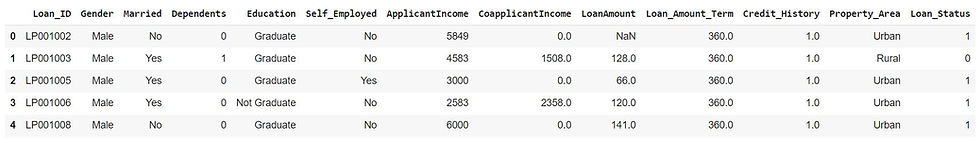top of page
• Hackers Realm

# Target/Mean Encoding for Categorical Attributes | Machine Learning | Python

Mean encoding, also known as target encoding, is a technique used to encode categorical attributes in machine learning models using python. It involves replacing each category with the mean target value of the corresponding target variable. The mean target value is calculated based on the training dataset.Target/Mean Encoding for Categorical Attributes

Mean encoding or target encoding can be effective in capturing the relationship between a categorical variable and the target variable. By encoding categorical attributes based on the target variable, it can capture valuable information that may improve the predictive power of the model.

You can watch the video-based tutorial with step by step explanation down below.

First we will have to load the data

```df = pd.read_csv('data/Loan Prediction Dataset.csv')
• We will read the CSV file 'Loan Prediction Dataset.csv' located in the 'data' directory and assign it to the DataFrame df using read_csv() function

• The head() method is called on the DataFrame df to display the first few rows of the modified DataFrameFirst few rows of Dataframe

## Convert the target value into label encoder

Next we will have to convert the target attribute values into label encoder

```df['Loan_Status'] = df['Loan_Status'].map({'Y':1, 'N':0})
• The above code snippet maps the values of the 'Loan_Status' column in the DataFrame. It assigns the value 1 to 'Y' (indicating loan approval) and the value 0 to 'N' (indicating loan rejection)

• The head() method is then used to display the first few rows of the updated DataFrame, allowing you to inspect the changesFirst few rows of Dataframe after label encoding

## Perform Target Encoding

Next we will utilize the TargetEncoder from the category_encoders library to perform target encoding on the 'Gender' and 'Dependents' columns in the DataFrame, using the 'Loan_Status' column as the target variable.

```from category_encoders import TargetEncoder
cols = ['Gender', 'Dependents']
target = 'Loan_Status'
for col in cols:
te = TargetEncoder()
# fit the data
te.fit(X=df[col], y=df[target])
# transform
values = te.transform(df[col])
df = pd.concat([df, values], axis=1)

• A TargetEncoder object is created and fitted to the 'Gender' and 'Dependents' columns using the target variable 'Loan_Status'

• The fit_transform() method is then used to transform the original categorical columns into their target-encoded representations

• The target-encoded values are stored in a new DataFrame called 'values'. The original DataFrame ('df') and the target-encoded values are concatenated column-wise using the pd.concat() function

• The column names for the target-encoded values are appended with '_encoded' to distinguish them from the original categorical columns

• Finally, the updated DataFrame is displayed using the head() method to view the changes made through target encodingFirst few rows of Dataframe after target encoding
• Here we are not able to see Female in Gender so let us just shuffle the data and display it again

`df.sample(frac=1).head(10)`
• This randomly shuffles the rows of the DataFrame df and then displays the first 10 rowsFirst few rows of Dataframe after shuffling
• We can see that for Female Gender type the average target value is 0.669643 and for Male Gender type the average target value is 0.693252

• For Dependent column with value 0 has average target value as 0.689855 , for value 1 the average target value is 0.647059 and for value 2 the average value is 0.752475

• Based on this we can easily predict the target value

• Similarly you can use the target encoding for all the categorical variables that you have in your dataset for creating new features. This will also improve the model performance

## Final Thoughts

• Target encoding provides a way to incorporate categorical attributes into machine learning models that typically require numerical input. It transforms categorical attributes into continuous numerical values, making it easier for models to process

• Target encoding has the risk of overfitting, especially when dealing with categories that have very few samples. Overfitting occurs when the encoding becomes too reliant on the target variable and fails to generalize well to unseen data. Regularization techniques like smoothing or noise addition can help mitigate this issue

• When working with imbalanced categories, where some categories have significantly more samples than others, target encoding may be biased towards the majority class. This can lead to misleading results. Techniques such as adding prior probabilities or considering stratified encodings can help address this problem

• Target encoding should be performed within the training data only to avoid data leakage. Calculating the mean target values should be based on the training set and then applied consistently to the validation or test sets. Otherwise, the encoding may introduce information from the validation or test sets into the training process, leading to overly optimistic performance estimates

• It is important to evaluate the impact of target encoding on the model's performance. Using cross-validation or other robust evaluation techniques can help assess the effectiveness of target encoding in improving model accuracy or other evaluation metrics

In this article we have explored how target encoding can be used to improve the performance of machine learning model. Overall, target encoding can be a powerful technique for encoding categorical attributes, but it requires careful implementation and evaluation to avoid potential pitfalls like overfitting, data leakage, and biased encoding. It is recommended to experiment with different encoding techniques and compare their performance to find the most suitable approach for your specific dataset and modeling task

Get the project notebook from here